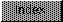```
There are three other parameters the scaling engine needs in order
answer queries for information:

OT_DeviceDPI            OT_PointHeight          OT_GlyphCode

The OT_DeviceDPI tag refers to the resolution of the output device.
The tag value's high word is the horizontal resolution and the low
word is the vertical resolution.  Both are unsigned words measured in
dots per inch.

The OT_PointHeight tag refers to the height of a typeface in points.
One point is approximately equal to 1/72 of an inch
(AGFA/Compugraphic defines the point to be 0.01383 inches).  For CG
typefaces, this height is the distance between baselines from one
line to the next (without any leading adjustments).  The point height
is represented as a fixed point, two's complement binary number, with
the point situated in the middle of a long word.  This means the
lower word is the fractional portion and the upper word the whole
number portion (the number covers the powers of two from 215 through
2-16).

For those who may not know, a two's complement number is a way of
representing negative numbers.  To find the two's complement of a
negative number, find the one's complement of the absolute value of
that number (change all the binary ones to zeros and all the zeros to
ones) and then add one to the result.  To change from two's
complement, subtract one from the two's complement number and find
the one's complement of the number.  For example,

Before conversion to two's complement, the absolute value of the one
byte decimal quantity -32 is represented as:

In binary         0010 0000  (\$20)
One's complement  1101 1111  (\$DF)
Add One           0000 0001  (\$01)
-----------------------------------
Two's complement  1110 0000  (\$E0)

So the number -32 is encoded as 1110 0000 or 0xE0 in hex.  Notice
that the high bit (the sign bit) of the encoded number is set if the
number is negative.  If the number is zero or greater, the high bit
is clear.  This procedure is independent of where the ``point'' is in
the negative number (the ``point'' in this case is the divider
between the whole portion of the number and the fractional portion).
When the computer adds one to the one's complement, it does not
consider where the ``point'' is in the one's complement, the computer
just treats the one's complement value as a whole integer.  For
example, to encode the decimal quantity -531/256 as a two byte, fixed
point, two's complement binary number where the point is situated in
the middle of the two bytes:

531/256 = -(2 + 19/256) = -2.13 in hex

In binary           0000 0010.0001 0011   (\$02.13 in hex, with the point)

One's complement    1111 1101 1110 1100   (\$FDEC  in hex ignore the point
from now on)
Add One             0000 0000 0000 0001   (\$0001)
----------------------------------------
Two's complement    1111 1101 1110 1101   (\$FDED)

Notice that the one added to the one's complement is in the 2-8 (=
1/256) column.

The OT_GlyphCode tag refers to the current glyph.  When an
application asks the scaling engine to rasterize a glyph, this is the
glyph the engine renders.  The scaling engine uses Unicode encoding
to represent glyphs.  Unicode is an international character encoding
standard that encompasses many of the world's national scripts in a
16-bit code space.  Conveniently, the Amiga's Latin-1, 8-bit
character set corresponds to the same glyphs as the Unicode standard.
To set the current glyph to a character from the Amiga character set,
use the same Latin-1 code, but pad it out to a 16-bit value.

Because the Compugraphic typefaces use their own character set, the
scaling engine in the bullet.library has to map the Unicode glyph
codes to Compugraphic glyph codes.  Note that the Unicode standard
encompasses many more glyphs than just the Latin-1 character set or
the Compugraphic character set, so many of the characters in the
Unicode set do not map to any glyphs in the Compugraphic set.  For
example, Unicode includes several Asian ideogram sets, that the
Compugraphic set does not.  The result is the vast majority of the
Unicode characters are not available using Compugraphic typefaces.
The Compugraphic character set covers roughly 400 glyphs.  For more
information on the UniCode standard, see the Unicode Consortium's
book The Unicode Standard, Worldwide Character Encoding published by